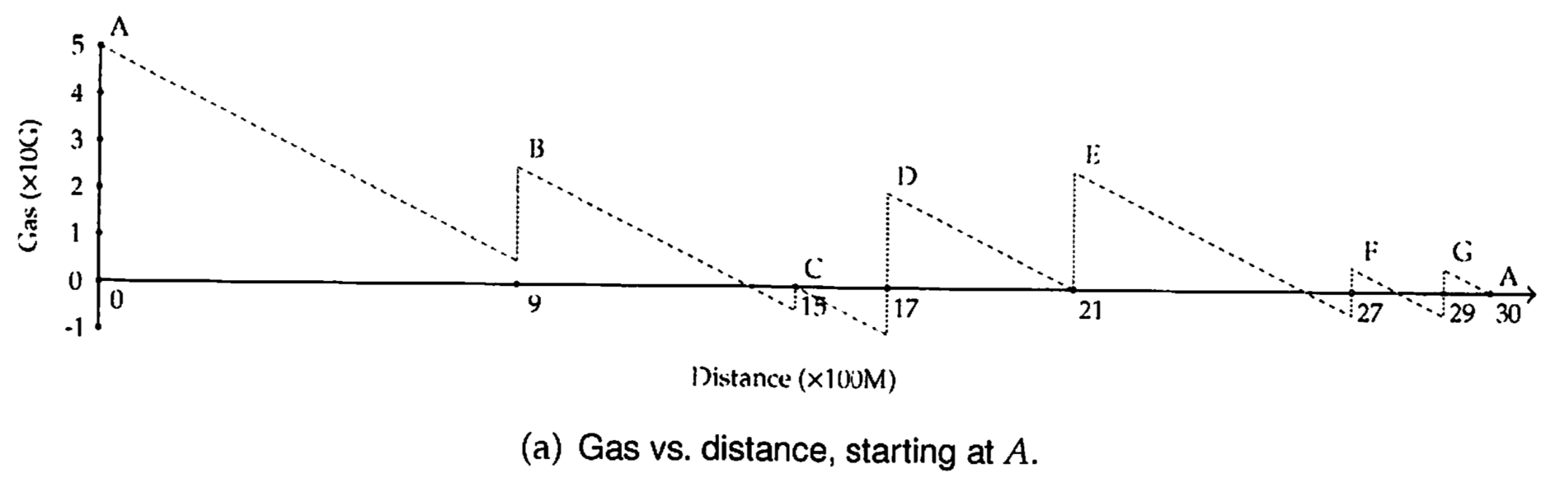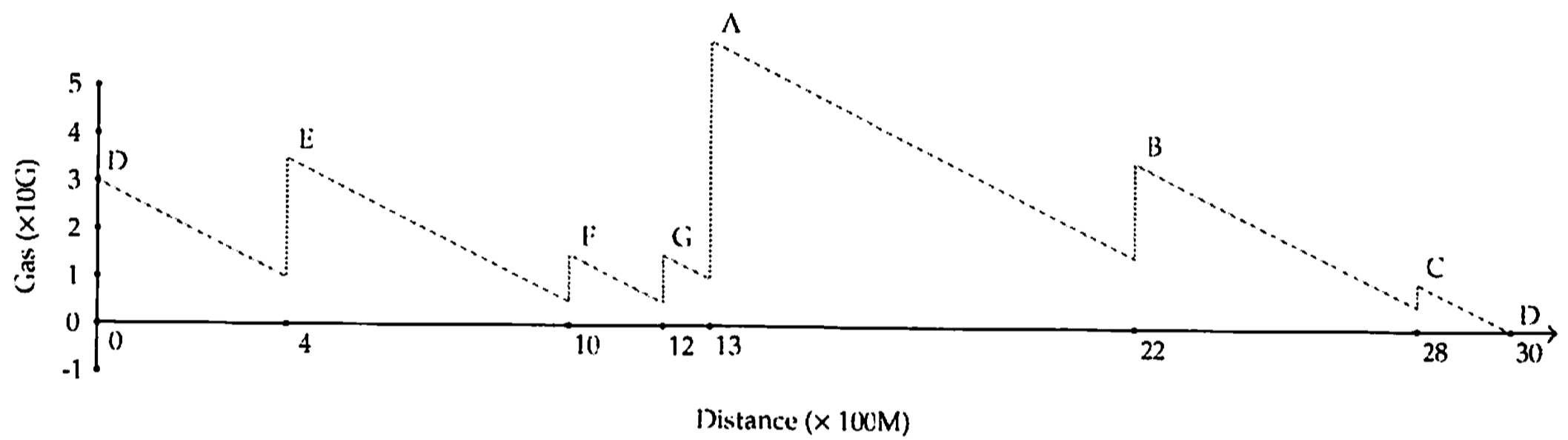# 134. Gas Station

Reference: LeetCode EPI 17.6
Difficulty: Medium

## Problem

There are N gas stations along a circular route, where the amount of gas at station i is gas[i].

You have a car with an unlimited gas tank and it costs cost[i] of gas to travel from station i to its next station (i + 1). You begin the journey with an empty tank at one of the gas stations.

Return the starting gas station’s index if you can travel around the circuit once in the clockwise direction, otherwise return -1.

Note:

• If there exists a solution, it is guaranteed to be unique.
• Both input arrays are non-empty and have the same length.
• Each element in the input arrays is a non-negative integer.

Example:

## Analysis

### Brute-Force

The idea of brute-force is to examine each single station (or city in EPI book):

• Choose the station as a starting point.
• Simulate the road trip to see if the following stations are reachable.

Time: $O(N^2)$
Space: $O(1)$

### Greedy

Proof by Contradiction: LeetCode Solution (if you have time)

The illustration in the EPI book helps understand the algorithm.

Note: The problem statement is a bit different, but the idea is the same. (Distance equals Gas Cost, City equals Station)We can see that at D we have the lowest gallon before refueling (negative gallon is allowed in the algorithm). Now take the station D as the starting point then we can have the optimal solution (if it exists).At last, check the starting point at last to see if such an ample station exists. If the gallon at last is negative which means we don’t have enough gallons in total to do the road trip (return -1).

Time: $O(N)$
Space: $O(1)$

CommentJunhao Wang
a software engineering cat